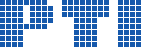Polish Information Processing Society# Annals of Computer Science and Information Systems, Volume 11

## Formalization of Pell's Equations in the Mizar System

### Marcin Acewicz, Karol Pąk

Citation: Proceedings of the 2017 Federated Conference on Computer Science and Information Systems, M. Ganzha, L. Maciaszek, M. Paprzycki (eds). ACSIS, Vol. 11, pages 223226 ()

Full text

Abstract. We present a case study on a formalization of a textbook theorem that is listed as \#39 at Freek Wiedijk's list of ``Top 100 mathematical theorems''. We focus on the formalization of the theorem that Pell's equation \$x^2-Dy^2 = 1\$ has infinitely many solutions in positive integers for a given non square natural number \$D\$.We present also a formalization of the theorem that based on the least fundamental solution of the equation we can simply calculate algebraically each remaining solution.

### References

1. A. Grabowski, “Efficient rough set theory merging,” Fundamenta Informaticae, vol. 135, no. 4, pp. 371–385, October 2014. http://dx.doi.org/10.3233/FI-2014-1129
2. K. Pąk, “Readable formalization of Euler’s partition theorem in Mizar,” in Intelligent Computer Mathematics – International Conference, CICM 2015, Washington, DC, USA, July 13–17, 2015, Proceedings, 2015. http://dx.doi.org/10.1007/978-3-319-20615-8_14 pp. 211–226.
3. G. Bancerek, C. Bylinski, A. Grabowski, A. Korniłowicz, R. Matuszewski, A. Naumowicz, K. Pąk, and J. Urban, “Mizar: State-of-the-art and beyond,” in Intelligent Computer Mathematics - International Conference, CICM 2015, ser. LNCS, vol. 9150. Springer, 2015. doi: 10.1007/978-3-319-20615-8_17 pp. 261–279.
4. W. Sierpiński, Elementary theory of numbers, A. Schinzel, Ed. Mathematical Institute of the Polisch Academy of Science, 1964.
5. J. Harrison, “The HOL Light system REFERENCE,” 2014, http://www.cl.cam.ac.uk/ jrh13/hol-light/reference.pdf.
6. N. D. Megill, “Metamath: A Computer Language for Pure Mathematics,” 2007, http://us.metamath.org/downloads/metamath.pdf.
7. G. Bancerek, “Cardinal arithmetics,” Formalized Mathematics, vol. 1, no. 3, pp. 543–547, 1990.